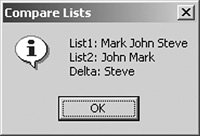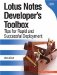# Compare Two Lists

This routine illustrates how to compare two fields or lists that contain multiple values. The resulting string or text list subsequently contains the differences between the two arrays.

How It Works

Using the @Replace statement, all elements in List1 that are found in List2 are replaced with a null string. The @trim() function removes all null strings from the result. The new value is then stored in the Result object. Using the @Prompt statement, the results are displayed to the user.

ImplementationExample 1

This first example illustrates how to sort static values. This example displays the contents of List1, List2, and the difference(s) between the lists. To implement this solution, insert the following statements in an action button formula, column formula, or the default value formula for a field, replacing the list values. The @Prompt statement is used for illustrative purposes and can be removed or updated as desired.

```List1 := "Mark" : "John" : "Steve";
List2 := "John" : "Mark";
Result := @Trim( @Replace(List1; List2; "") );

@Prompt([Ok]; "Compare Lists";
"List1: " + @Implode(List1) + @NewLine +
"List2: " + @Implode(List2) + @NewLine +
"Delta: " + @Implode(Result))
```

When the button is clicked, the message shown in Figure 14.1 is displayed. Notice that the correct result is produced even when each list contains values sorted in a different order.

Figure 14.1. Example of comparing two arraysImplementationExample 2

The second example illustrates how to sort dynamic values. Here, List1 and List2 reference fields on a document, and Delta contains the difference between the two lists. To implement this solution, insert the following statements in an action button formula, column formula, or the default value formula for a field. Replace FIELDA and FIELDB with valid document field names. The @Prompt statement is used for illustrative purposes and can be removed or updated as desired.

```List1 := FIELDA;
List2 := FIELDB;
Result := @Trim( @Replace(List1; List2; "") );

@Prompt([Ok]; "Compare Lists";
"List1: " + @Implode(List1) + @NewLine +
"List2: " + @Implode(List2) + @NewLine +
"Delta: " + @Implode(Result))
```

Tip

Select Allow multiple values if using this approach to set a field on a form and the result may contain multiple values. This option is located on tab 1 of the field properties dialog.Lotus Notes Developers Toolbox: Tips for Rapid and Successful Deployment
ISBN: 0132214482
EAN: 2147483647
Year: N/A
Pages: 293
Authors: Mark Elliott

Similar book on Amazon

Flylib.com © 2008-2020.
If you may any questions please contact us: flylib@qtcs.net